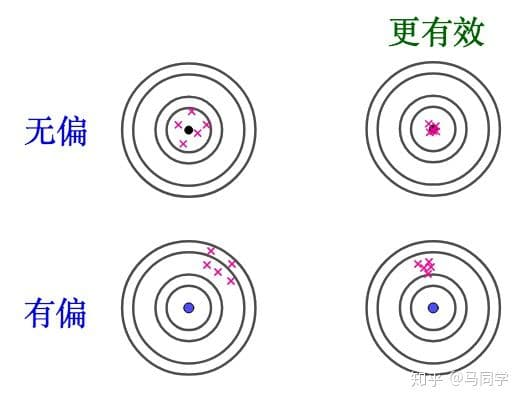# 估计的评价标准

\begin{align*} \newcommand{\dif}{\mathop{}\!\mathrm{d}} \newcommand{\p}{\partial} \newcommand{\Cov}{\text{Cov}}\\ \newcommand{\Mse}{\mathrm{Mse}} \end{align*}

# 无偏性准则

定义{：success}$$E(\overline{X})=E(\frac{1}{n} \sum_{i=1}^n X_i)=\frac{1}{n}\sum_{i=1}^n E(X_i)=\mu$$

$$E(S^2)=\sigma^2$$

$$E(B_2)=\frac{n-1}{n} E(S^2)=\frac{n-1}{n}\sigma^2\\ \lim_{n\rightarrow \infty} E(B_2)=\sigma^2$$

# 有效性准则

定义

# 均方误差准则

定义